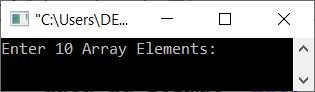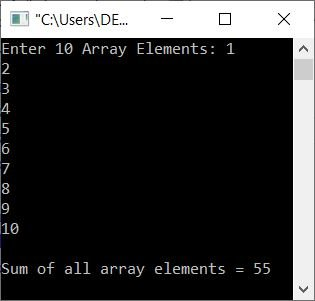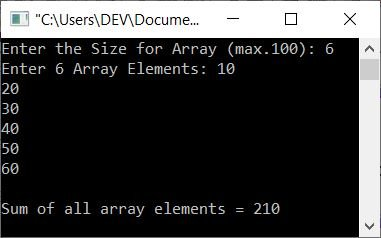# C++ Program to Find and Print the Sum of Array Elements

This article provides a program in C++ to find and print the sum of all elements available in an array. Here, the elements of the array must be entered by the user at run-time.

## Find the sum of an array's elements

The question is: write a program in C++ that finds and prints the sum of all elements or numbers in a given array. The following program is its answer:

```#include<iostream>

using namespace std;
int main()
{
int arr, i, sum=0;
cout<<"Enter 10 Array Elements: ";
for(i=0; i<10; i++)
cin>>arr[i];
for(i=0; i<10; i++)
sum = sum+arr[i];
cout<<"\nSum of all array elements = "<<sum;
cout<<endl;
return 0;
}```

Here is the initial output produced by the above C++ program on finding the sum of all elements of an array entered by the user:Now enter any ten numbers one by one and press the ENTER key to find and print the sum of all elements, as shown in the snapshot given below:Since there is a limitation to the above program, That is, the user is only allowed to enter the 10 elements. Therefore, let's modify this program and create another one that allows the user to define the size of the array along with its elements:

```#include<iostream>

using namespace std;
int main()
{
int tot, arr, i, sum=0;
cout<<"Enter the Size for Array (max.100): ";
cin>>tot;
cout<<"Enter "<<tot<<" Array Elements: ";
for(i=0; i<tot; i++)
{
cin>>arr[i];
sum = sum+arr[i];
}
cout<<"\nSum of all array elements = "<<sum;
cout<<endl;
return 0;
}```

Here is its sample run with user input, 6 as size, and 10, 20, 30, 40, 50, and 60 as six array elements:C++ Quiz

« Previous Program Next Program »